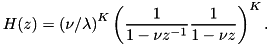A Survey of Gaussian Convolution Algorithms
Alvarez-Mazorra Gaussian convolution

A first-order recursive filter approximation, computed in-place. More...

## Detailed Description

A first-order recursive filter approximation, computed in-place.

This code implements Alvarez and Mazorra's recursive filter approximation of Gaussian convolution. The Gaussian is approximated by a cascade of first-order recursive filters,References
• Alvarez, Mazorra, "Signal and Image Restoration using Shock Filters and Anisotropic Diffusion," SIAM J. on Numerical Analysis, vol. 31, no. 2, pp. 590-605, 1994. http://www.jstor.org/stable/2158018

## Functions

void am_gaussian_conv (num *dest, const num *src, long N, long stride, double sigma, int K, num tol, int use_adjusted_q)
Gaussian convolution with Alvarez-Mazorra. More...

void am_gaussian_conv_image (num *dest, const num *src, int width, int height, int num_channels, num sigma, int K, num tol, int use_adjusted_q)
2D Gaussian convolution with Alvarez-Mazorra More...

## Function Documentation

 void am_gaussian_conv ( num * dest, const num * src, long N, long stride, double sigma, int K, num tol, int use_adjusted_q )

Gaussian convolution with Alvarez-Mazorra.

Parameters
 dest output convolved data src input data, modified in-place if src = dest N number of elements stride stride between successive samples sigma standard deviation of the Gaussian in pixels K number of passes (larger implies better accuracy) tol accuracy in evaluating left boundary sum use_adjusted_q if nonzero, use proposed regression for q

Implements the fast approximate Gaussian convolution algorithm of Alvarez and Mazorra, where the Gaussian is approximated by K passes of a first- order causal filter and a first-order anticausal filter,Boundaries are handled with half-sample symmetric extension, and tol specifies the accuracy for approximating an infinite sum on the left boundary.

Gaussian convolution is approached as approximating the heat equation and each timestep is performed with an efficient recursive computation. Using more steps yields a more accurate approximation of the Gaussian. Reasonable values for the parameters are K = 4, tol = 1e-3.

The convolution can be performed in-place by setting src = dest (the source array is overwritten with the result).

Definition at line 81 of file gaussian_conv_am.c.

 void am_gaussian_conv_image ( num * dest, const num * src, int width, int height, int num_channels, num sigma, int K, num tol, int use_adjusted_q )

2D Gaussian convolution with Alvarez-Mazorra

Parameters
 dest output convolved data src input, modified in-place if src = dest width,height,num_channels the image dimensions sigma Gaussian standard deviation K number of passes tol accuracy for left boundary sum use_adjusted_q if nonzero, use proposed q

Similar to am_gaussian_conv(), this routine approximates 2D Gaussian convolution with Alvarez-Mazorra recursive filtering.

The convolution can be performed in-place by setting src = dest (the source array is overwritten with the result).

Definition at line 159 of file gaussian_conv_am.c.